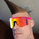TradingView3949 views
The Omega Ratio is a risk-return performance measure of an investment asset, portfolio, or strategy. It is defined as the probability-weighted ratio, of gains versus losses for some threshold return target. The ratio is an alternative for the widely used Sharpe ratio and is based on information the Sharpe ratio discards.

█ OVERVIEW

As we have mentioned many times, stock market returns are usually not normally distributed. Therefore the models that assume a normal distribution of returns may provide us with misleading information. The Omega Ratio improves upon the common normality assumption among other risk-return ratios by taking into account the distribution as a whole.

█ CONCEPTS

Two distributions with the same mean and variance, would according to the most commonly used Sharpe Ratio suggest that the underlying assets of the distribution offer the same risk-return ratio. But as we have mentioned in our Moments indicator, variance and standard deviation are not a sufficient measure of risk in the stock market since other shape features of a distribution like skewness and excess kurtosis come into play. Omega Ratio tackles this problem by employing all four Moments of the distribution and therefore taking into account the differences in the shape features of the distributions.

Another important feature of the Omega Ratio is that it does not require any estimation but is rather calculated directly from the observed data. This gives it an advantage over standard statistical estimators that require estimation of parameters and are therefore sampling uncertainty in its calculations.

█ WAYS TO USE THIS INDICATOR

Omega calculates a probability-adjusted ratio of gains to losses, relative to the Minimum Acceptable Return (MAR). This means that at a given MAR using the simple rule of preferring more to less, an asset with a higher value of Omega is preferable to one with a lower value. The indicator displays the values of Omega at increasing levels of MARs and creating the so-called Omega Curve.
Knowing this one can compare Omega Curves of different assets and decide which is preferable given the MAR of your strategy. The indicator plots two Omega Curves. One for the on chart symbol and another for the off chart symbol that u can use for comparison.

When comparing curves of different assets make sure their trading days are the same in order to ensure the same period for the Omega calculations.

Value interpretation:

Omega<1 will indicate that the risk outweighs the reward and therefore there are more excess negative returns than positive.
Omega>1 will indicate that the reward outweighs the risk and that there are more excess positive returns than negative.
Omega=1 will indicate that the minimum acceptable return equals the mean return of an asset. And that the probability of gain is equal to the probability of loss.

█ FEATURES

• "Low-Risk security" lets you select the security that you want to use as a benchmark for Omega calculations.
• "Omega Period" is the size of the sample that is used for the calculations.
• “Increments” is the number of Minimal Acceptable Return levels the calculation is carried on.
• “Other Symbol” lets you select the source of the second curve.
• “Color Settings” you can set the color for each curve.

Open-source Skript

Ganz im Sinne von TradingView hat der Autor dieses Skripts es als Open-Source veröffentlicht, damit Trader es verstehen und überprüfen können. Ein Hoch auf den Autor! Sie können es kostenlos verwenden, aber die Wiederverwendung dieses Codes in einer Publikation unterliegt den Hausregeln. Sie können das Skript den Favoriten hinzufügen, um es auf dem Chart zu verwenden.

Möchten Sie dieses Skript auf einem Chart verwenden?
Our Website🅱🅰🐷: https://bapigindicator.com

Sign up on our website to get access to our indicators.

Join Our Discord for further discussions: https://discord.gg/RwuAK9MV

## KommentareAnother great indicator from powb team
Thanks bali and pig for making the best indiactor for retails like us !
Antworten500 is never enough at times! So, here is even more. You know what to do... Thank you for embracing the spirit of TV's true intentions. :)
AntwortenI trust you will ensure these coins are put to proper use, wink-wink. I admire voluntary contributions to the community more than you know!
Antworten